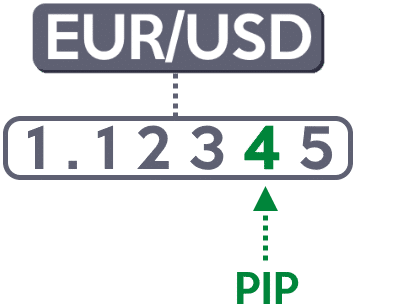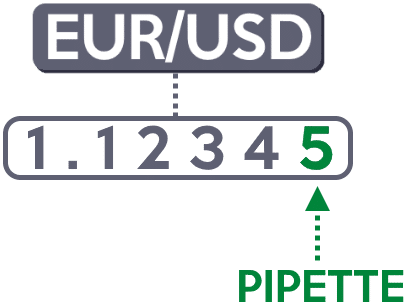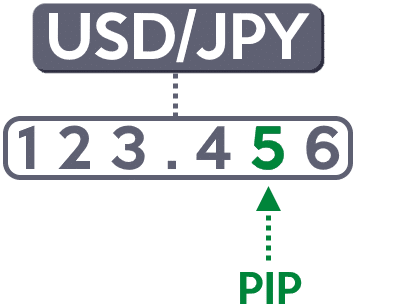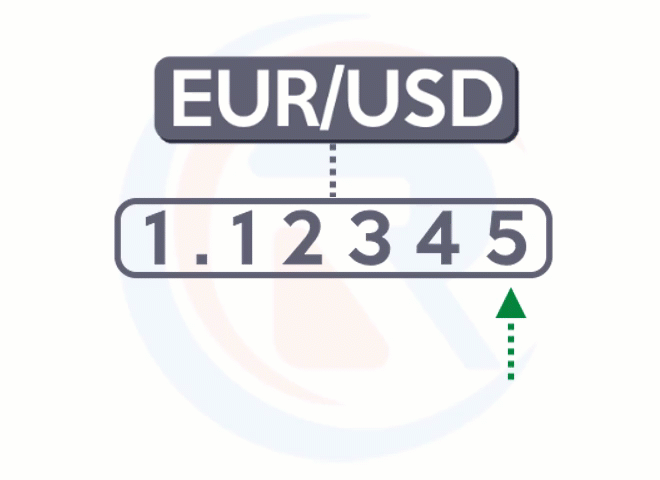Text

# #1: What is a pip

Lesson 1

## What is a pip

A pip refers to “point in percentage”.

But how do you know where a pip is?

Here’s how…

For pairs without JPY in it, 1 pip is on the 4th decimal place of the Forex pair:However…

For pairs with JPY in it, 1 pip is on the 2nd decimal place of the Forex pair:A pipette is also known as a fractional pip.

It’s a decimal place away from a pip.

For pairs without JPY in it, 1 pipette is on the 5th decimal place of the Forex pairFor pairs with JPY, 1 pipette is on the 3rd decimal place of the Forex pairPut it all together and here’s what it looks like:So…

If EUR/USD moves from 1.1234 to 1.1235, that’s an increase of 1 pip.

If EUR/USD moves from 1.1234 to 1.0234, that’s a decrease of 1000 pips.

If GBP/JPY moves from 123.45 to 123.55, that’s an increase of 10 pips.

If GBP/JPY moves from 123.45 to 120.45, that’s a decrease of 300 pips.

Are you getting it so far?

Next…

## How to calculate the value of a pip?

The way to calculate the value of a pip is simple:

1. Determine the value per pip of this currency pair you’re trading
2. Determine the spot rate between your account currency and the quote currency (the “second half” of the currency pair)
3. Convert value per pip from the quote currency to your account currency

Example 1:

You go long on 100,000 units of EUR/USD.

So…

If EUR/USD increases by 1 pip, how does it affect your P&L (in CAD)?

Step 1: Determine the value per pip of this currency pair you’re trading

EUR is the base currency (the “first half” of the currency pair), and USD is the quote currency (the “second half” of the currency pair).

To determine the value per pip, let’s look at the quote currency.

If you’re long 100,000 units of EUR/USD…

The value per pip is 0.0001 x 100,000 = 10 USD per pip.

Step 2: Determine the spot rate between your account currency and the quote currency

The quote currency is in USD.

Thus, look at the spot rate of USD/CAD.

Step 3: Convert value per pip from the quote currency to your account currency

Let’s assume the spot rate of USD/CAD = 1.3000.

Thus, 1.3 x 10 = 13 CAD.

This tells you that every 1 pip of movement in EUR/USD is worth \$13 CAD to you.

Example 2:

Your trading account is in SGD and you long 10,000 units of GBP/JPY.

If GBP/JPY moves by 1 pip, what’s the impact on your P&L (in SGD)?

Step 1: Determine the value per pip of this currency pair you’re trading

The GBP is the base currency and the JPY is the quote currency.

Look at the quote currency to determine the value per pip.

Since you’re long 10,000 units of GBP/JPY…

The value per pip is 0.01 x 10,000 = 100 JPY per pip.

Step 2: Determine the spot rate between your account currency and the quote currency

The quote currency is JPY.

So, look at the spot rate of SGD/JPY.

Step 3: Convert value per pip from the quote currency to your account currency

Let’s assume the spot rate of SGD/JPY = 79.00.

If 79 JPY is worth 1 SGD, how much SGD can you get with 100 JPY?

So…

100 ÷ 79 = 1.26 SGD.

This means that every 1 pip movement in GBP/JPY is worth \$1.26 SGD to you.

Now…

Don’t worry if this is too much work for you.

You can use this FREE pip-value calculator that I’ve created this for you.